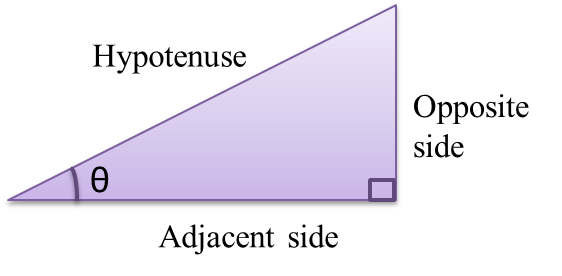# 9.1.1 Values of Sine, Cosine and Tangent of an Angle (Part 1)

9.1 Values of Sine, Cosine and Tangent of an Angle

(A) Sine, Cosine and Tangent for Right-Angled Triangles$\overline{)\begin{array}{l}\text{}\mathrm{sin}\theta =\frac{\text{opposites side}}{\text{hypotenuse}}\text{}\\ \\ \text{cos}\theta =\frac{\text{adjacent side}}{\text{hypotenuse}}\\ \\ \text{tan}\theta =\frac{\text{opposites side}}{\text{adjacent side}}\end{array}}$

(B) Values of Sin θ, Cos θ and Tan θ in First Quadrant of the Unit CircleIn first quadrant, P is a point on the circumference of the unit circle with centre O, θ is the angle between the radius OP and the positive x-axis. From the diagram,
$\begin{array}{l}\text{(a) sin}\theta =\frac{PQ}{OP}=y\\ \text{(b) cos}\theta =\frac{OQ}{OP}=x\\ \text{(c) tan}\theta =\frac{PQ}{OQ}=\frac{y}{x}\\ \\ Therefore,\\ \overline{)\begin{array}{l}\text{}\\ \text{sin}\theta =y\text{-coordinate}\\ \text{cos}\theta =x\text{-coordinate}\\ \text{tan}\theta =\frac{y\text{-coordinate}}{x\text{-coordinate}}\end{array}}\end{array}$

(C) A Cartesian plane is divided into four parts called quadrants by the x-axis and the y-axis. The quadrants are named as first quadrant, second quadrant, third quadrant and fourth quadrant in the anticlockwise direction.Quick recall: All – Add Sine – Sugar Tangent – To Cosine – Coffee

Example:
Determine whether the value of each of the following is positive or negative.
(a) sin 105o (b) cos 75o (c) tan 305o (d) sin 50o
(e) cos 160o (f) tan 220o (g) cos 260o (h) cos 350o

Solution:

(a) sin 105o is positive because 90o < 105o< 180o (in second quadrant).

(b) cos 75o is positive because 0o < 75o< 90o (in first quadrant).

(c) tan 305o is negative because 270o < 305o< 360o (in fourth quadrant).

(d) sin 50o is positive because 0o < 50o< 90o (in first quadrant).

(e) cos 160o is negative because 90o < 160< 180o (in second quadrant).

(f) tan 220o is positive because 180o < 220o< 270o (in third quadrant).

(g) cos 260o is negative because 180o < 260o< 270o (in third quadrant).

(h) cos 350o is positive because 270o < 350o< 360o (in fourth quadrant).